## 5.1.3 Basic Measure Theory Definitions

This section briefly indicates how to define volume in a metric space. This provides a basis for defining concepts such as integrals or probability densities. Measure theory is an advanced mathematical topic that is well beyond the scope of this book; however, it is worthwhile to briefly introduce some of the basic definitions because they sometimes arise in sampling-based planning.

Measure can be considered as a function that produces real values for subsets of a metric space,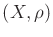. Ideally, we would like to produce a nonnegative value,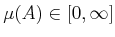, for any subset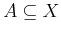. Unfortunately, due to the Banach-Tarski paradox, if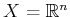, there are some subsets for which trying to assign volume leads to a contradiction. Ifis finite, this cannot happen. Therefore, it is hard to visualize the problem; see  for a construction of the bizarre nonmeasurable sets. Due to this problem, a workaround was developed by defining a collection of subsets that avoids the paradoxical sets. A collection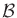of subsets ofis called a sigma algebra if the following axioms are satisfied:

1. The empty set is in.
2. If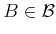, then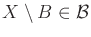.
3. For any collection of a countable number of sets in, their union must also be in.
Note that the last two conditions together imply that the intersection of a countable number of sets inis also in. The sets inare called the measurable sets.

A nice sigma algebra, called the Borel sets, can be formed from any metric spaceas follows. Start with the set of all open balls in. These are the sets of the form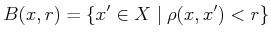(5.13)

for any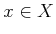and any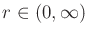. From the open balls, the Borel setsare the sets that can be constructed from these open balls by using the sigma algebra axioms. For example, an open square in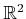is inbecause it can be constructed as the union of a countable number of balls (infinitely many are needed because the curved balls must converge to covering the straight square edges). By using Borel sets, the nastiness of nonmeasurable sets is safely avoided.

Example 5..9 (Borel Sets)   A simple example ofcan be constructed for. The open balls are just the set of all open intervals,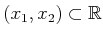, for any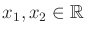such that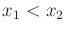.Using, a measure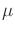is now defined as a function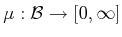such that the measure axioms are satisfied:

1. For the empty set,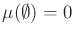.
2. For any collection,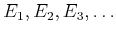, of a countable (possibly finite) number of pairwise disjoint, measurable sets, let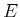denote their union. The measuremust satisfy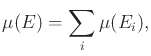(5.14)

in whichcounts over the whole collection.

Example 5..10 (Lebesgue Measure)   The most common and important measure is the Lebesgue measure, which becomes the standard notions of length in, area in, and volume in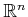for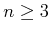. One important concept with Lebesgue measure is the existence of sets of measure zero. For any countable set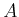, the Lebesgue measure yields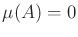. For example, what is the total length of the point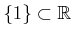? The length of any single point must be zero. To satisfy the measure axioms, sets such as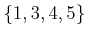must also have measure zero. Even infinite subsets such as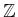and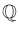have measure zero in. If the dimension of a set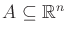is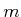for some integer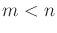, then, according to the Lebesgue measure on. For example, the set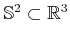has measure zero because the sphere has no volume. However, if the measure space is restricted to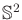and then the surface area is defined, then nonzero measure is obtained.Example 5..11 (The Counting Measure)   Ifis finite, then the counting measure can be defined. In this case, the measure can be defined over the entire power set of. For any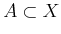, the counting measure yields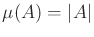, the number of elements in. Verify that this satisfies the measure axioms.Example 5..12 (Probability Measure)   Measure theory even unifies discrete and continuous probability theory. The measurecan be defined to yield probability mass. The probability axioms (see Section 9.1.2) are consistent with the measure axioms, which therefore yield a measure space. The integrals and sums needed to define expectations of random variables for continuous and discrete cases, respectively, unify into a single measure-theoretic integral.Measure theory can be used to define very general notions of integration that are much more powerful than the Riemann integral that is learned in classical calculus. One of the most important concepts is the Lebesgue integral. Instead of being limited to partitioning the domain of integration into intervals, virtually any partition into measurable sets can be used. Its definition requires the notion of a measurable function to ensure that the function domain is partitioned into measurable sets. For further study, see [346,546,836].

Steven M LaValle 2020-08-14